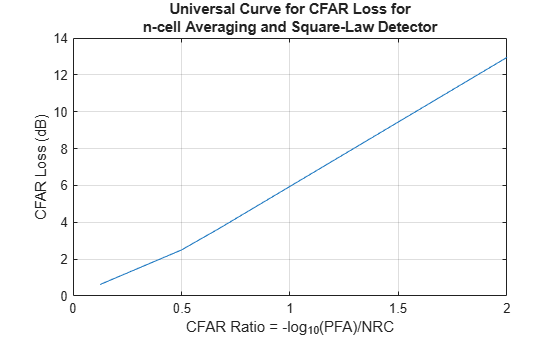# cfarloss

Loss due to constant false alarm rate (CFAR) adaptive processing

## Syntax

``LCFAR = cfarloss(PFA,NRC)``
``LCFAR = cfarloss(PFA,NRC,Name,Value)``

## Description

example

````LCFAR = cfarloss(PFA,NRC)` computes approximated CFAR loss, `LCFAR`, in dB for the probability of false alarm, PFA, and number of reference cells, NRC, that you specify. The function calculates loss for the cell-averaging (CA) CFAR method and a square-law detector based on the Gregers-Hansen's universal CFAR loss curve. ```
````LCFAR = cfarloss(PFA,NRC,Name,Value)` specifies additional options using name-value arguments. For example, ```LCFAR = cfarloss(1e-8,4:4:64,'Method','CA')``` computes approximate loss using the CA CFAR process.. You can specify multiple name-value arguments. ```

## Examples

collapse all

Calculate the CFAR loss for an n-cell averaging and a square-law detector. Assume the numbers of reference cells from `4–64` and the probability of false alarm of `1e-8`.

```PFA = 1e-8; NRC = 4:4:64; LCFAR = cfarloss(PFA,NRC);```

Plot the resulting loss vs CFAR ratio. The CFAR ratio is calculated using the equation, X = -${\mathrm{log}}_{10}$(`PFA`)/`NRC`.

```plot(-log10(PFA)./NRC,LCFAR) grid on; xlabel('CFAR Ratio = -log_{10}(PFA)/NRC'); ylabel('CFAR Loss (dB)'); title({'Universal Curve for CFAR Loss for',... 'n-cell Averaging and Square-Law Detector'});```## Input Arguments

collapse all

Probability of false alarm, specified as a positive scalar in the range [`1e-15,1e-3`] or as a length-K vector with each element in the range [`1e-15,1e-3`] .

Number of reference cells used in CFAR processing, specified as a positive scalar or length-K vector.

### Name-Value Pair Arguments

Specify optional comma-separated pairs of `Name,Value` arguments. `Name` is the argument name and `Value` is the corresponding value. `Name` must appear inside quotes. You can specify several name and value pair arguments in any order as `Name1,Value1,...,NameN,ValueN`.

Example: ```LCFAR = cfarloss(1e-8,4:4:64,'DetectorType','Log')```

Type of CFAR process, specified as a either `'CA'` for cell-averaging process or `'GOCA'` for greatest-of cell-averaging process.

Example: `'Method','GOCA'`

Type of detector in use, specified as either `'SquareLaw'`, `'Linear'`, or `'Log'`.

Example: `'DetectorType','Linear'`

## Output Arguments

collapse all

CFAR loss, returned as a K-element vector in dB.

## Extended Capabilities

### C/C++ Code GenerationGenerate C and C++ code using MATLAB® Coder™.